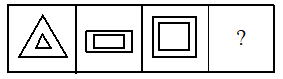In each of the following question, there are three problem figures followed by a question mark (?) for the fourth one. There exists relationship between the first two problems figures. A similar relationship should exist between the third and fourth figure. Find the one from the answer figures that currency replaces the questions mark.

# In each of the following question, there are three problem figures followed by a question mark (?) for the fourth one. There exists relationship between the first two problems figures. A similar relationship should exist between the third and fourth figure. Find the one from the answer figures that currency replaces the questions mark.1. A
2. B
3. C
4. D

Fill Out the Form for Expert Academic Guidance!l

+91

Live ClassesBooksTest SeriesSelf Learning

Verify OTP Code (required)

### Solution:

Three sided figure converted to four sided. Similarly four sided figure convert to five sided.+91

Live ClassesBooksTest SeriesSelf Learning

Verify OTP Code (required)